# Methods & Suitability of Depreciation Calculations

Several depreciation methods have been coming out for the O Level examinations such as the Revaluation and Units of Production method. We will discuss the two main methods commonly used – the Straight Line Method (SLM) and the Reducing Balance Method (RBM).

### 1. Straight Line Method

Calculation of Depreciation:

Depreciation can be calculated if the following items of information are available:

• Cost of the asset includes all expenses incurred for freight, carriage, and installation costs.
• Scrap or residual value of the asset. I call it the garang-guni value – that amount we estimate the trash collection uncle will take when he collects from your place.
• Useful life (not the physical life) of asset.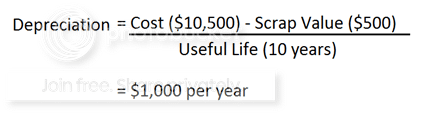### 2. Reducing (Diminishing) Balance Method

Calculation of Depreciation:

Depreciation = Rate x Net Book Value (NBV)

where

NBV = Cost* – Provision for (Accumulated) Depreciation

In Year 2,

#### Depreciation expense using RBM = 10% x (10,000 – 1,000)

EXAMPLE

Using two different methods, a \$10,000 asset with no scrap value at a depreciation rate of 10% will result in a different depreciation expense and net book value of the asset from Year 2 onwards.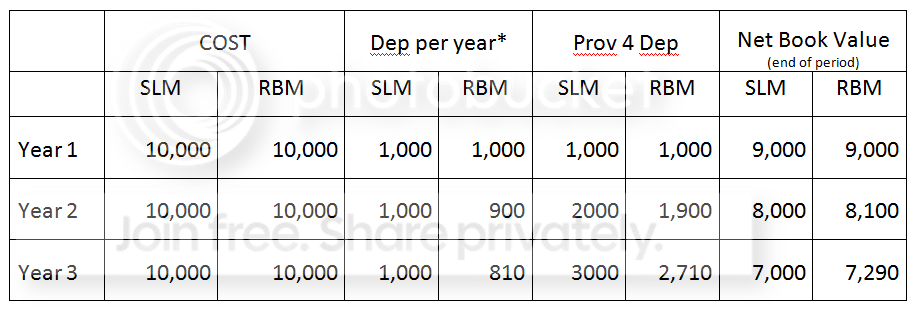· Straight Line = 10% X \$10,000 = \$1,000 per year

· Reducing Balance = 10% x Net Book Value Asset at the end of the previous period

Often, the depreciation rate used in RBM is usually between two and three times greater than under the SLM. Besides the depreciation charges, there are also the maintenance and repairs costs element and these running costs usually increases with age.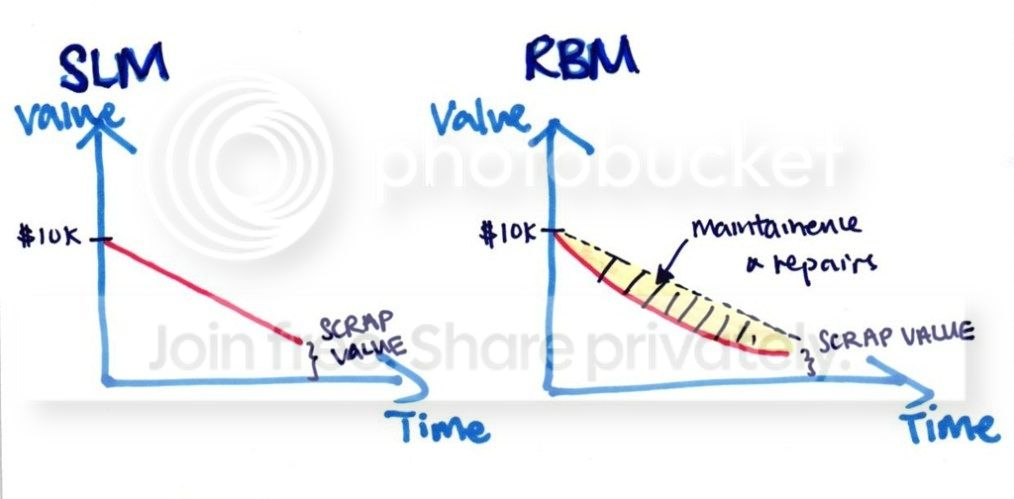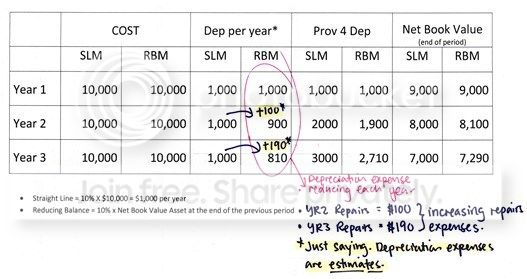#### In Reducing Balance Method,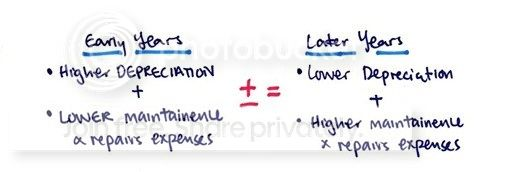## Suitability of Methods

How suitable the depreciation method is on an asset depends on how well it reflects the benefits the business derives from the used of assets.

Straight line method is more suitable for fixed assets that generates a more constant benefits such as Furniture and Fittings. it is fairer and more realistic.

Reducing balance method is used for fixed assets which depreciate more in the early years and in later years due to the efficiency of the fixed asset is higher in the early year. Therefore, reducing balance method used for assets such as motor vehicle and computers.

Revaluation Method (for loose tools and cutlery) – see notes below on Materiality Concept

Other Methods of Providing Depreciation:

Machine Hour Rate Method, Sum of Year’s Digit Method, Production unit method or Depletion method etc.

## Why Different Methods?

To adhere to the Matching principle, because the revenue earned must be matched with the expenses incurred (depreciation) from the fixed asset that was used to generate that income within the same accounting period.

Prudence/Conservatism principle is adhered because the fixed asset is valued based on their net book value after accumulated depreciation so that assets and profits will not be overstated.

Materiality Concept is applied when the amount is insignificant. Loose tools which are immaterial in dollar amount may be written off as expense in one period, even though it may have a useful life of more than an accounting period.

## SUMMARY OF DIFFERENCES